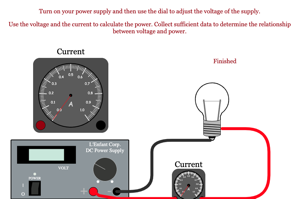Programs for Programs for Ohm's Law
Below are all the programs that might be used with this topic. Click on "See Resources" to see if there are any student directions, lab sheets or other materials that have been created for this program.
Simple Circuits

Lab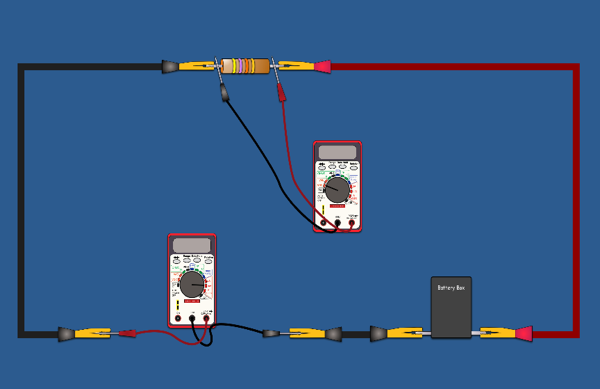Power of Light Bulb Lab

Lab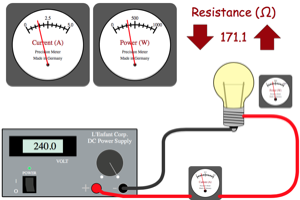Ohm's Law with Analog Meters

Lab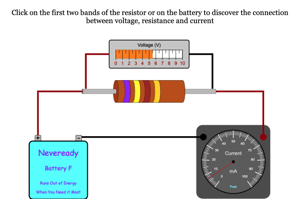Current in Wire Problem

Homework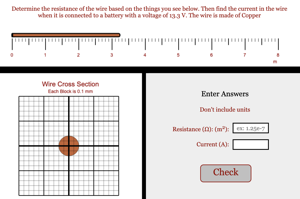Finding Battery Voltage Simple

Homework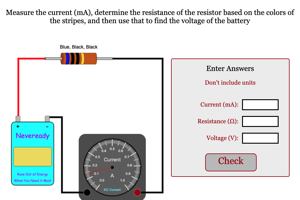Finding Battery Voltage Medium

Homework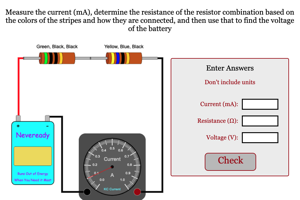Finding Battery Voltage Harder

Homework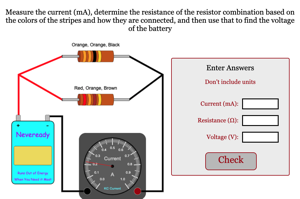Finding Battery Voltage Very Hard

Homework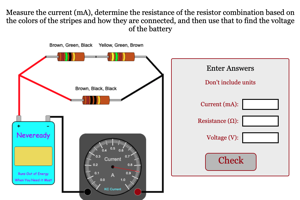Finding Battery Voltage Hardest

Homework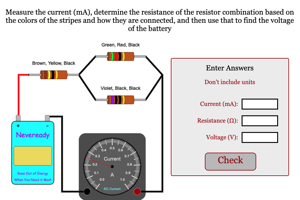Multimeter Function Challenge

Game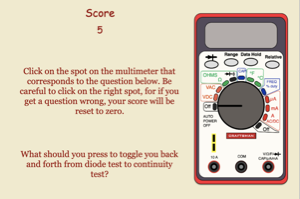Finding Battery Power Simple

Homework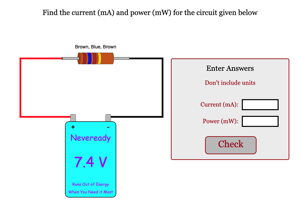Ohm's Law with Predictions Lab

Lab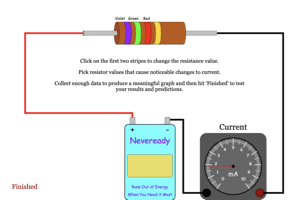Battery, Light, Meter Problem

Homework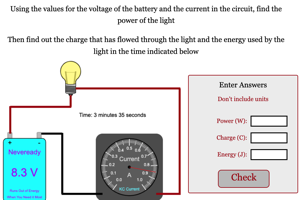Power of Light with Prediction Lab

Lab